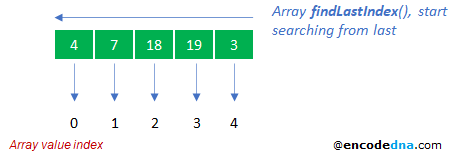﻿ How to find an element from Last in Array using JavaScript

# How to find an element from Last in Array using JavaScript

There are two new methods in JavaScript that you can use to find an element from last in an Array. The first method is findLast() and the second method is findLastIndex().

👉  Don’t forget to read the conclusion.

In its previous edition, ECMAScript introduced the find() and "findIndex()" methods, using which you can find an element (based on a condition) "from the beginning" of an array or starting from the “1st value” (or the first element that matched a condition). I have explained these two methods in detail here.

Now let’s see how to find values from the last in an array in JavaScript.

## Using findLast() method

The findLast() method is an iterative method. That is, it loops through each value or item in an array starting from the last element and looks for a value based on a condition.

For example,

```<script>
const arr = [18, 7, 11, 19, 3];
document.write('the value is ' + arr.findLast(item => item > 6));
</script>```

I have a list of numbers in an array and I want find values that are "greater than" 6, starting from the last. So, the first matching value it found is number 19.

## Find the Last Even number in an Array

Here’s another example, where I want to extract or find the even number in the array starting from the last element.

```<script>
const arr = [4, 7, 18, 19, 3];
document.write('the value is ' + arr.findLast(item => item % 2 === 0));
</script>```

You can apply the method to an JSON object. For example,

```<script>
const student = '[{"subject":"science", "marks": 87}, ' +
'{"subject":"math", "marks": 78}, ' +
'{"subject":"english", "marks": 72}, ' +
'{"subject":"sanskrit", "marks": 91}]';

let arr = JSON.parse(student);
arr = arr.findLast(student => student.marks < 80);
document.write ('The value is ' + arr.subject);
</script>```

## Using findLastIndex() method

The findLastIndex() will not return the value (based on a condition), but it would return the index of the element (or value).

👉  Remember: It will start looking for values from the last value in the array. However, it will return the "index" of the first matching value. Array "index" always starts from "0" (from the beginning).

```<script>
const arr = [4, 7, 18, 19, 3];
document.write('the value is ' + arr[arr.findLastIndex(item => item > 6)]);
</script>```The first matching value is "19". findLastIndex() returns the index value 3. So, arr will return the value 19.

👉  Note: If it doesn’t find a match, "findLastIndex()" will return -1. For example,

```<script>
const arr = [4, 1.8, 3.2, 2, 3];
document.write('the index is ' + arr.findLastIndex(item => item > 6));  // Output: -1
</script>```
Conclusion

In many ways, the methods findLast and findLastIndex are useful. No doubt about it. However, many say it was unnecessary because there were alternative methods previously to find an element from last in an array.

For example, I can use the reverse() and find() methods together to do the same. See this…

```<script>
const arr = [18, 7, 11, 19, 3];
document.write (arr.reverse().find(item => item > 6));   // Output: 19
</script>```

The result is similar to the 1st example that I have shared here in this post, where I have used "findLast()" method.

Happy coding .